# 15. 机器学习 - 支持向量机Hi, 你好。我是茶桁。

## 逻辑回归预测心脏病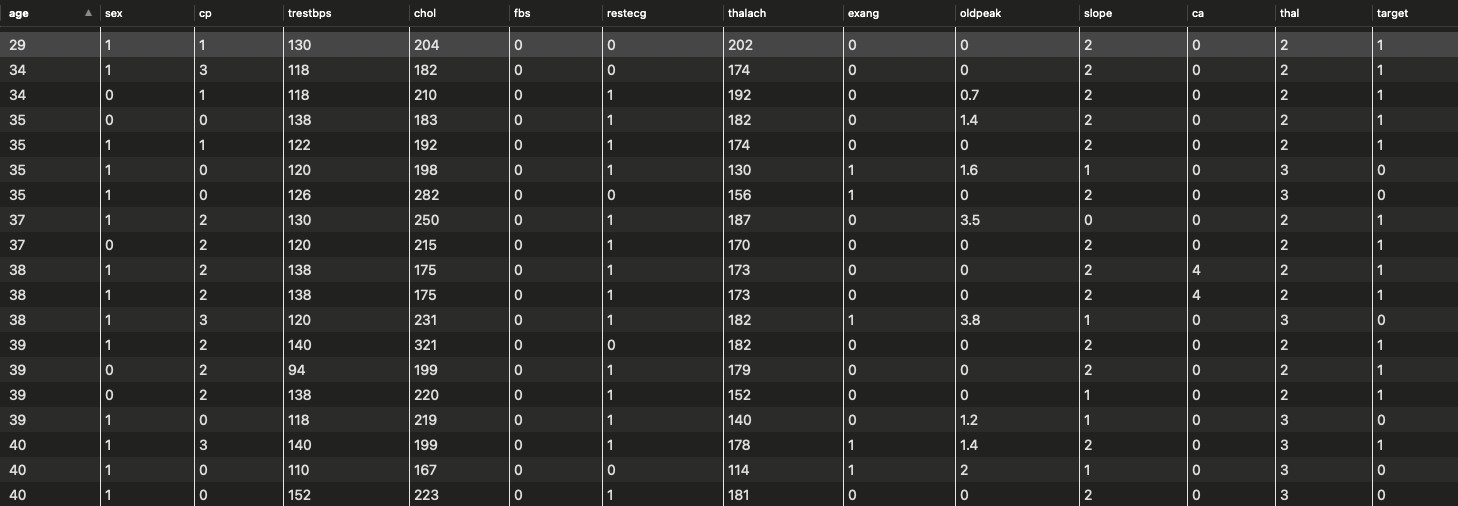## 支持向量机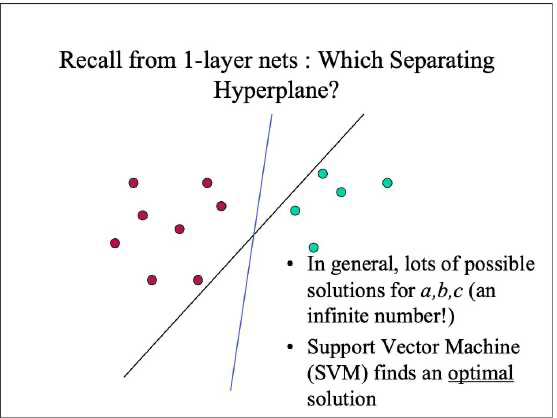\begin{align*} min_{w, b}\frac{1}{2}||w||^2 \end{align*}

$y_i(w \cdot x_i+b) \ge 1$

w 是超平面的法向量，b 是截距项，yi 是样本 xi 的标签，也就是 +1 或者 -1。

$L(w,b,a) = \frac{1}{2}||w||^2 - \sum_{i=1}^Na_i[y_i(w\cdot x_i +b) - 1]$

$a_i[y_i(w\cdot x_i + b)-1] = 0$

$w = \sum_{i=1}^Na_iy_ix_i \\ sum_{i=1}^N a_iy_i = 0$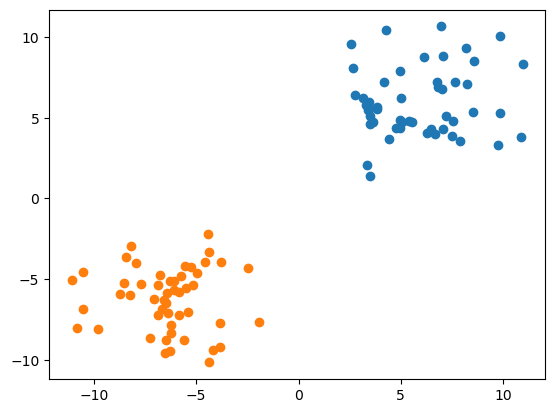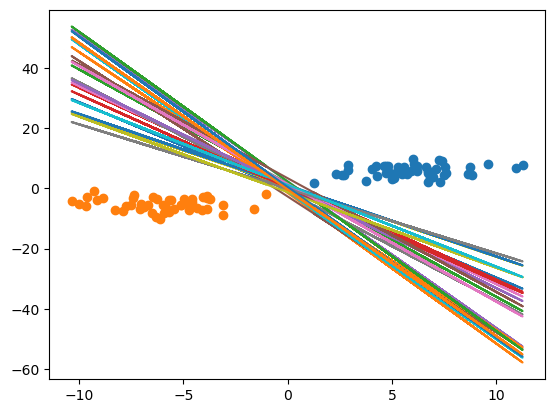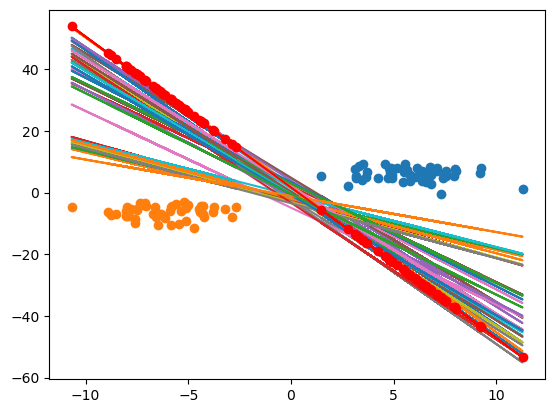## 核函数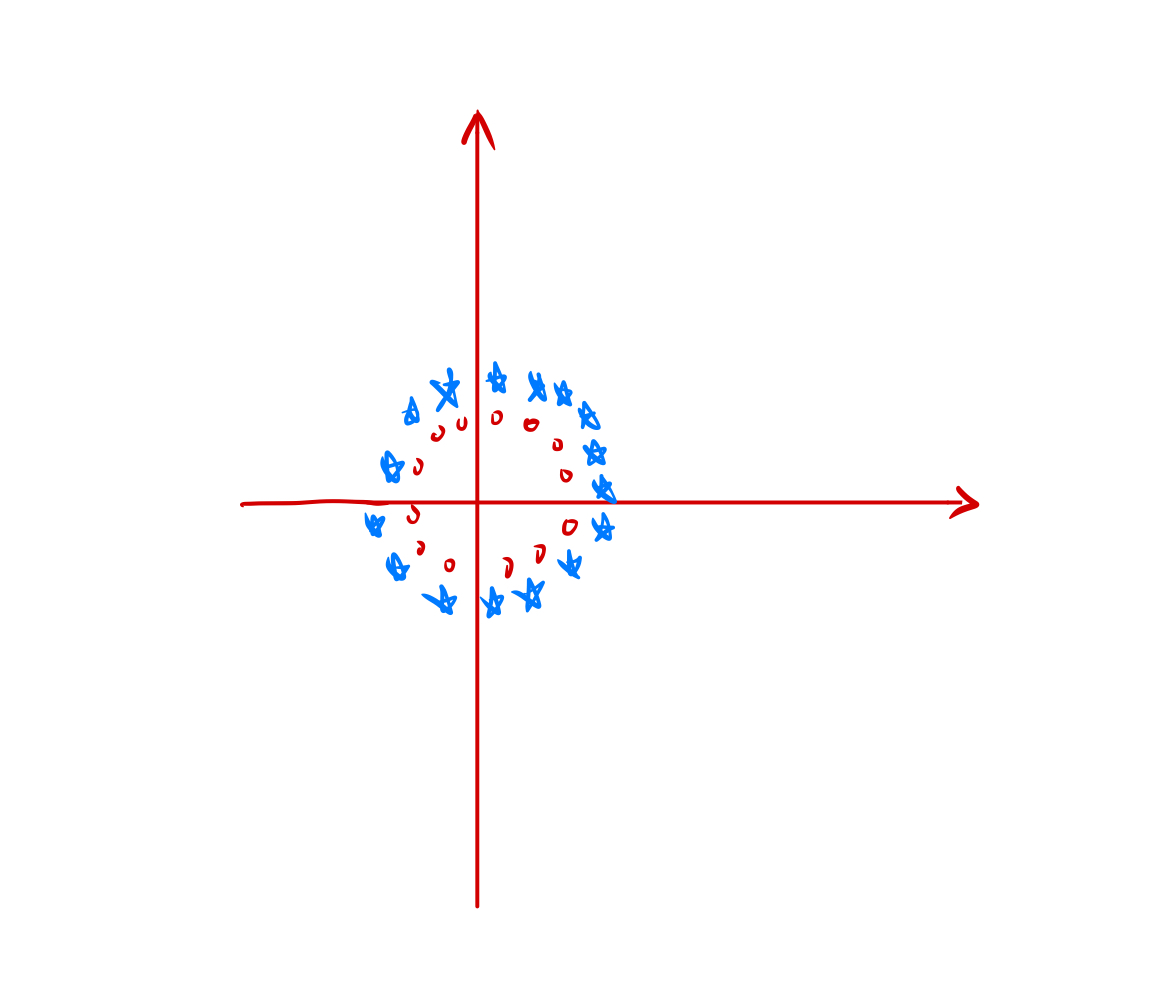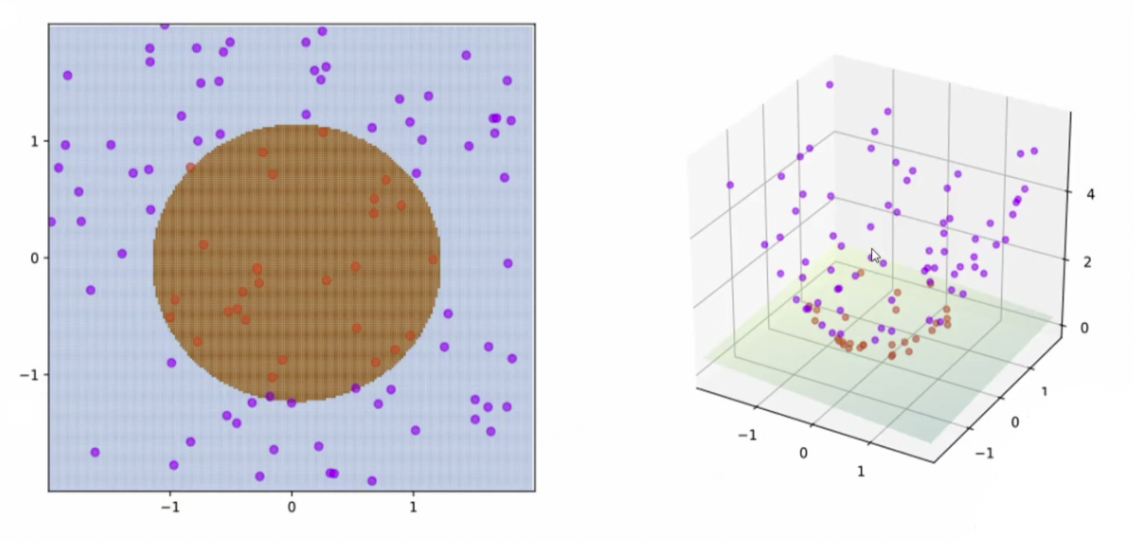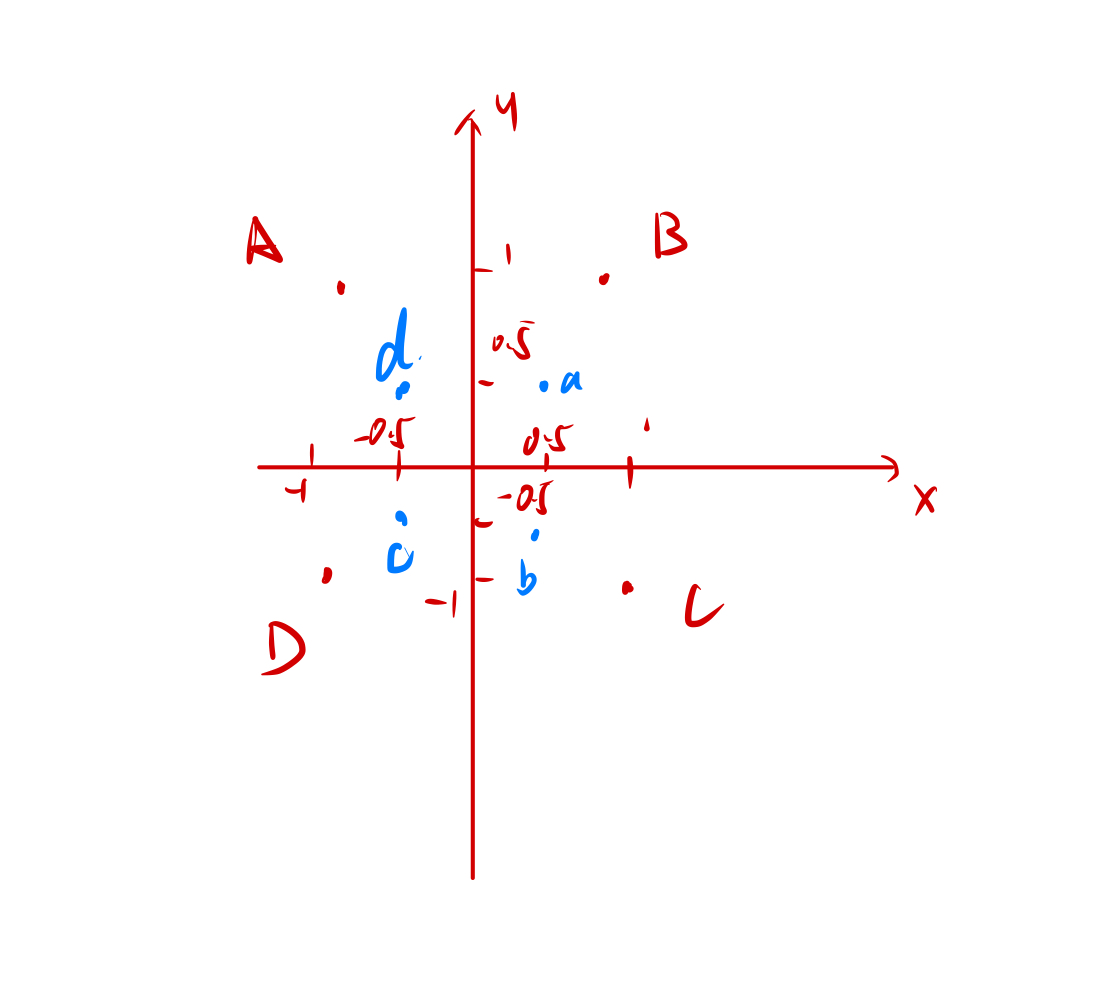\begin{align*} f(x) => \begin{Bmatrix} x^2 \\ y^2 \end{Bmatrix} \end{align*}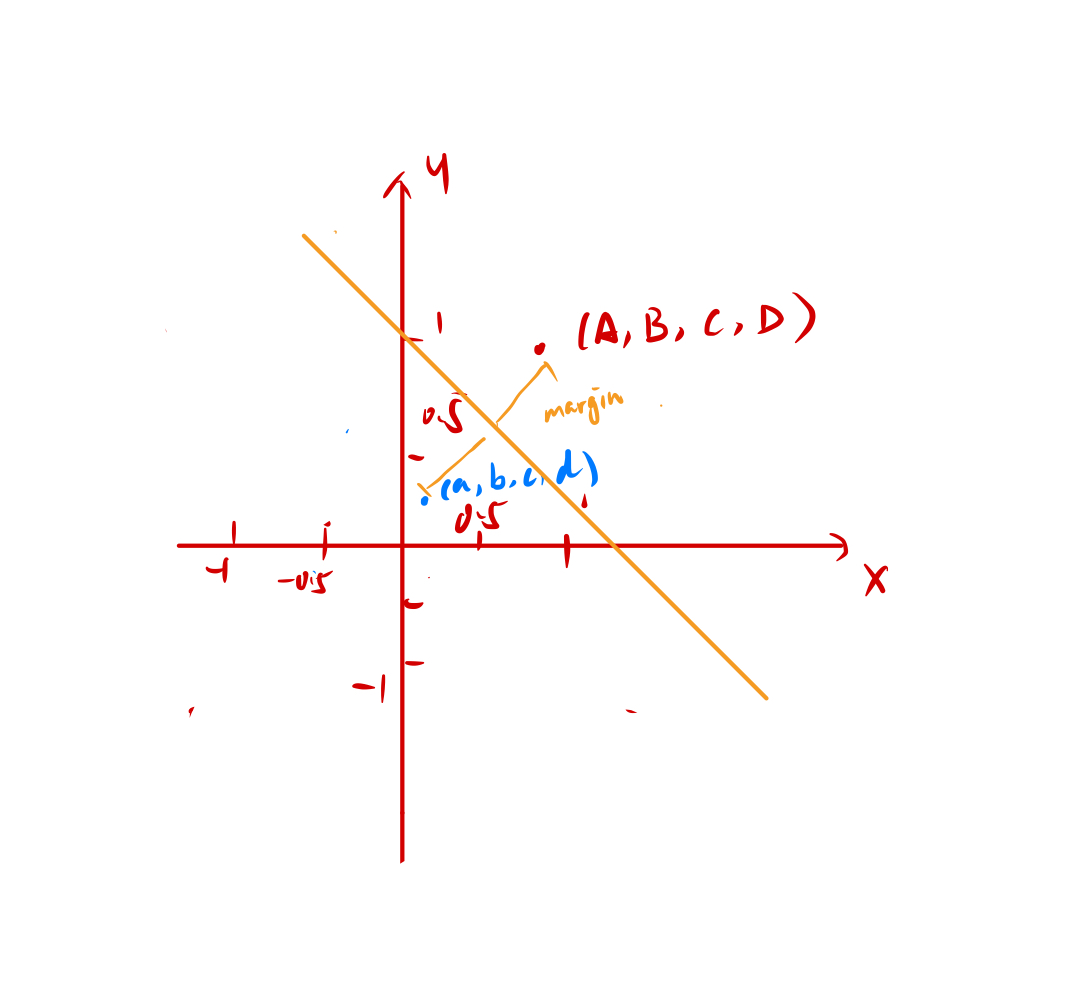$\varphi (x) = \begin{bmatrix} x \\ x^2 \\ x^3 \end{bmatrix}$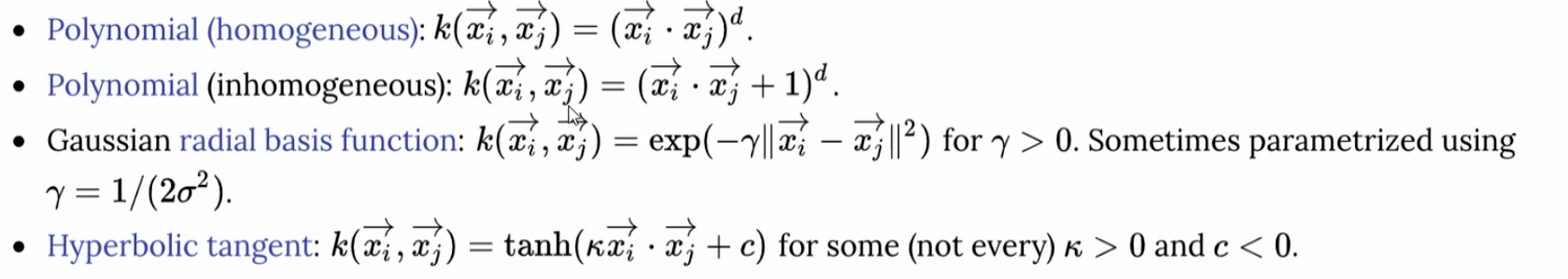SVM 其实也有弊端，当数据量很复杂的时候，现有的核函数就没有作用了。因为它会失效，所以我们需要很多的人工分析，整个效率很低。

SVM 因为要做各种升维，当数据量比较大的时候，计算量非常的复杂，计算需求量非常的大。Hivan Du

2023-10-30

2023-11-25